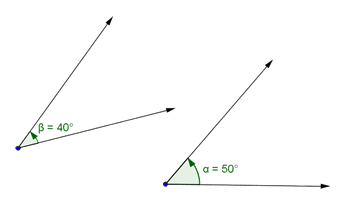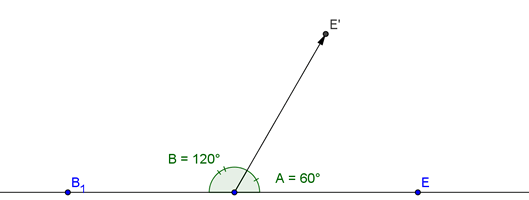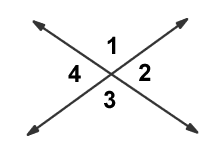# Special Types of Angles

Two angles may share a certain relationship that will be useful in solving a geometric problem. Determining that a pair of angles is complementary, supplementary, or vertical may be useful in determining other unknown angles.

### Complementary Angles

As you may know, two angles are complementary if the sum of their degree measures equals 90 degrees. Two angles, measuring 50 degrees and 40 degrees, are complementary because their sum is 90 degrees, as shown in the diagram below:Using this knowledge, we can begin to solve for unknown angles:

### Sample A

If the degree measure of two complementary angles are in the ratio of 1:14, find the degree measure of the smallest angle.

Solution:

Let x equal the degree measure of the smallest angle.

Then x + 14x = 90 degrees.

Why do we equate x + 14x to 90 degrees? This is done because we are dealing with complementary angles. That means the sum is 90 degrees. We called the smaller angle x, so the larger one must be 14x because we know it's 14 times larger. Therefore, the small angle (x) plus the larger angle (14x) must equal 90 degrees (because they are complementary).

We now simply solve for x:

x + 14x = 90 degrees
15x = 90 degrees
x = 90/15
x = 6

The smaller angle measures x degrees, so it is a 6 degree angle. The larger one is 14 times x, so it is 6*14 = 84 degrees. It may be important to remember that the problem specifically asked for the smaller angle, so make sure you give the answer the problem is looking for!

### Supplementary Angles

These are similar to complementary angles, except their sum is 180 degrees. Often you will see supplementary angles created in a ray shoots out from a flat line, such as below:Sample B

If the degree measure of an angle is 30 degrees greater than twice the degree measure of its supplementary angle, what is the degree measure of the angle?

Solution:

Notice that this question is about supplementary angles NOT complementary angles. That means they sum to 180 degrees.

Let d = the degree measure of the angle.

Then, of course, 180 degrees - d = the degree measure of the supplement of the angle. Is that clear? To say it another way, the supplement is whatever we have to add to d to equal 180.

We now set up an equation keeping in mind the word TWICE.

d = 2(180 degrees - d) + 30

Wow... where did that equation come from? Let's look at it step by step. The problem starts by saying "the degree measure of an angle is," and that's where the "d =" part comes from. Then it says "30 degrees greater than," which explains the "+30." The last part says "Twice the degree measure of its supplementary angle," which means "2*(180-d)."

We now solve for d:

d = 360 degrees - 2d + 30
d + 2d = 360 degrees + 30
3d = 360 degrees + 30
3d = 390 degrees
d = 130 degrees

### Vertical Angles

In the two examples above, we played with complementary and supplementary angles. In this section, we are going to deal with vertical angles and supplementary angles in a different way.

What is a vertical angle anyway?

Vertical angles are opposite pairs of congruent (or equal) angles that are made when 2 lines intersect (cross at a point). In the figure below, opposite angles 1 and 3 (and also opposite angles 2 and 4) are called vertical angles based on the definition given above.NOTE: Angles 1 and 2 are supplementary angles, because they add up to 180 degrees. You'll also notice that (2 and 3) are a pair of supplementary angles, as are (3 and 4) and (4 and 1). There's 4 sets of supplementary angles.

Let us say that the measure of angle 1 = 80. Since angle 1 = angle 3, then the measure of angle 3 is also 80. That's because we know vertical angles (across from each other) always have the exact same measure.

Suppose we were told that the measure of angle 2 = 25. Since angle 2 = angle 4, then angle 4 = 25.

### Sample C

The measure of angle 2 = x + 4. Find the measure of angle 4.

Solution:

Since angle 2 = angle 4 (because they are vertical angles), then the measure of angle 4 = x + 4.### Sample D

The measure of angle 1 = y - 5. Find the measure of angle 3.

Solution:

Since angle 1 = angle 3, (because they are vertical angles), then the measure of angle 3 = y - 5.### Sample E

Let us say that the measure of angle 1 = 40. What is the measure of angle 2?

Solution:

Since 1 & 2 are supplementary, the measure of angle 2 = 180 degrees - the measure of angle 1

The measure of angle 2 = 180 degrees - 40
The measure of angle 2 = 140 degree

We can check to make sure it is true. To check, we add 140 + the given angle ( 40 ). If we get get a sum of 180, then we are right.

LOOK:

140 + 40 = 180

180 = 180...It checks!!

Provided by Mr. Feliz